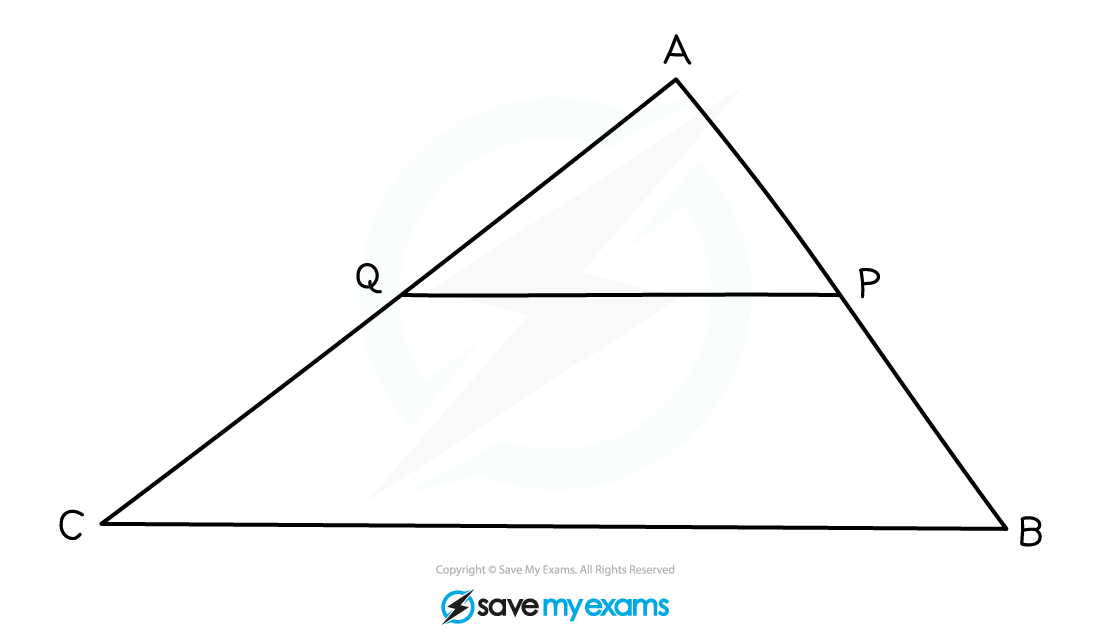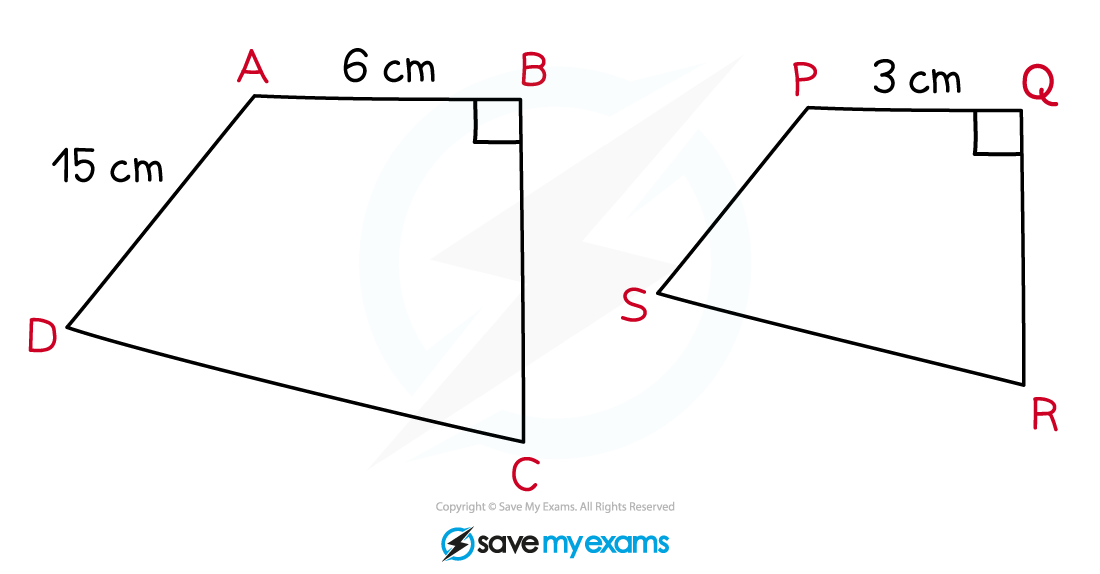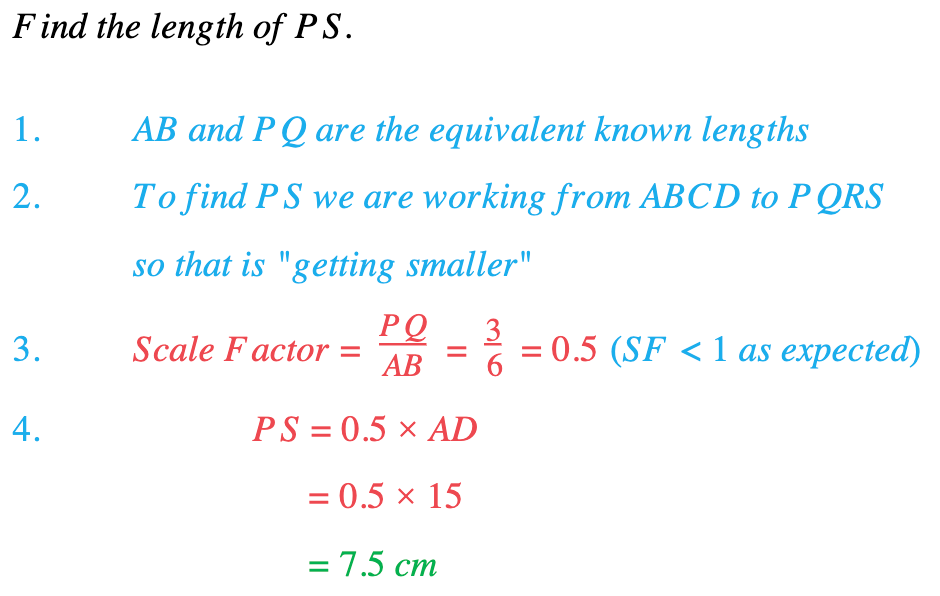# Edexcel IGCSE Maths 复习笔记 4.17.1 Similarity - Lengths

Edexcel IGCSE Maths 复习笔记 4.17.1 Similarity - Lengths

#### What are similar shapes?

• Two shapes are mathematically similar if one is an enlargement of the other
• Equivalent angles in the two shapes will be equal
• Equivalent lengths in the two shapes will be in the same ratio and are linked by a scale factor (which you will normally have to find)

#### Working with similar shapes

1. Identify equivalent known lengths
2. Establish direction (getting bigger or smaller?)
3. Find scale factor = Second Length ÷ First Length(Check that SF > 1 if getting bigger and SF < 1 if getting smaller)
4. Use scale factor to find length

#### Exam Tip

If similar shapes overlap on the diagram (or are not clear) draw them separately!For example, in this diagram the triangles ABC and APQ are similar:So we would redraw them separately before we start:#### Worked Example